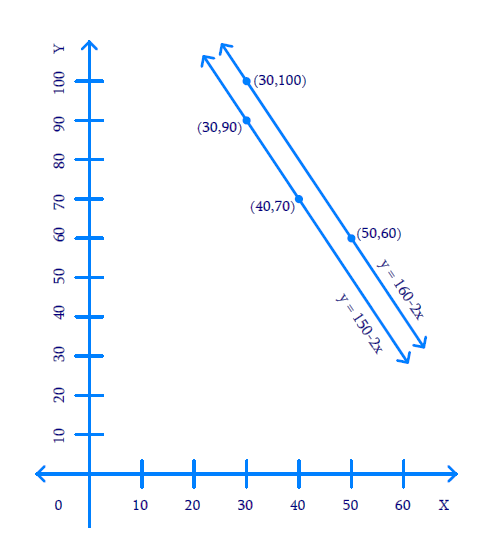# Ex.3.1 Q3 Pair of Linear Equations in Two Variables Solution - NCERT Maths Class 10

Go back to  'Ex.3.1'

## Question

The cost of $$2 \,\rm{kg}$$ of apples and $$1 \,\rm{kg}$$ of grapes on a day was found to be ₹ $$160$$ After a month, the cost of $$4\,\rm{kg}$$ of apples and $$2\,\rm{kg}$$ of grapes is ₹ $$300$$ Represent the situation algebraically and geometrically.

Video Solution
Pair Of Linear Equations In Two Variables
Ex 3.1 | Question 3

## Text Solution

What is Known?

(i) Cost of $$2 \,\rm{kg}$$of apples and $$1 \,\rm{kg}$$ of grapes is ₹ $$160$$

(ii) Cost of $$4 \,\rm{kg}$$ of apples and $$2 \,\rm{kg}$$ of grapes is ₹ $$300.$$

What is Unknown?

Represent the situation geometrically and algebraically.

Reasoning:

Assuming the cost of $$1 \,\rm{kg}$$ apples as ₹ $$x$$ and the cost of $$1 \,\rm{kg}$$ grapes as ₹ $$y,$$ two linear equations can be formed for the above situation.

Steps:

Let the cost of $$1 \,\rm{kg}$$ of apples be $$x$$ and cost of $$1 \,\rm{kg}$$ of grapes be $$y$$

Cost kg $$2 \,\rm{kg}$$ apples and $$1 \,\rm{kg}$$ of grapes is ₹ $$160.$$

Mathematically,

$2x + y = 160$

Also, cost kg $$4 \,\rm{kg}$$ apples and $$2 \,\rm{kg}$$ of grapes is ₹ $$300.$$

Mathematically,

\begin{align}4x + 2y &= 300\\2(2x + y) &= 300\\2x\, + \,y\,& = \,150\end{align}

Algebraic representation where $$x$$ and $$y$$ are the cost of $$1 \,\rm{kg}$$ apple and $$1 \,\rm{kg}$$ grapes respectively.

\begin{align}2x + y &= 160 \,\dots(1)\\2x + y& = 150\,\dots(2)\end{align}

Therefore, the algebraic representation is for equation $$1$$ is:

\begin{align}2x + y &= 160\\y &= 160-2x\end{align}

And, the algebraic representation is for equation $$2$$ is:

\begin{align}2x + y& = 150\\y &= 150-2x\end{align}

Let us represent these equations graphically. For this, we need at least two solutions for each equation. We give these solutions in table shown below.

 $$x$$ $$50$$ $$30$$ $$y = 160-2x$$ $$60$$ $$100$$

 $$x$$ $$30$$ $$40$$ $$y = 150-2x$$ $$90$$ $$70$$

The graphical representation is as follows.Unit $$= 1\, \rm{cm} =$$$$10$$

Since the lines are parallel hence no Solution

Learn from the best math teachers and top your exams

• Live one on one classroom and doubt clearing
• Practice worksheets in and after class for conceptual clarity
• Personalized curriculum to keep up with school